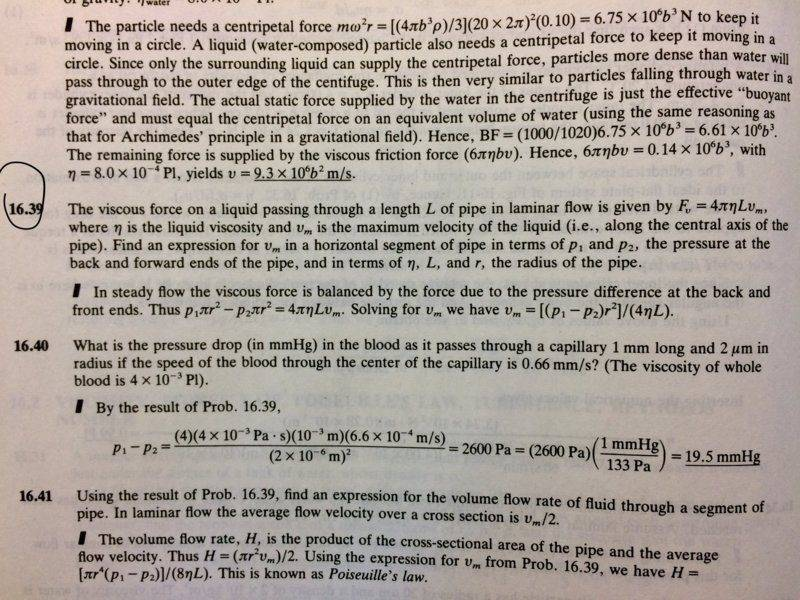# Writing Force equation in terms of pressure and area

## Homework Statement

Problem 16.39
[/B]
The viscous force of a liquid in laminar flow through length L is given by ##F_v=4\pi\etaL v_m## where ##\eta## is liquid viscosity and ##v_m## is maximum velocity of the fluid. Find an expression for ##v_m## in terms of p1, p2 (pressure at each end) and in terms of ##\eta , L, r## (r is radius of pipe)

*I'm not solving the whole problem, but I have a question about force.##F=p*A##

## The Attempt at a Solution

I look at the key and see that they are equating ##F_v## with the pressure difference. Can it just be one pressure?
Is there a difference between representing force as pressure times area as they are (##F=p * A##), versus when one or both of the variables is changing? ( ##F=\Delta p * A## or ##F=p* \Delta A ##). It makes sense to me if both variables are not changing. But if they changes, does it mean the force is changing as well, so we should write the equation as ##\Delta F##?

Thank you!

#### Attachments

Chestermiller
Mentor
F is the frictional tangential force at the wall, acting opposite to the direction of the fluid velocity.

F is the frictional tangential force at the wall, acting opposite to the direction of the fluid velocity.
Thank you, but I was just wondering what would be the difference between the force represented by ##pA## versus ##\Delta pA## or ##p\Delta A## . I know that the unit remains the same regardless but still, what would happen if one of the variables is changing. My question is not specific to the problem posted above.

Chestermiller
Mentor
Thank you, but I was just wondering what would be the difference between the force represented by ##pA## versus ##\Delta pA## or ##p\Delta A## . I know that the unit remains the same regardless but still, what would happen if one of the variables is changing. My question is not specific to the problem posted above.
If you know the pressure at a surface and the area of that surface, do you know how to get the force on the surface? Also, do you know how to perform a force balance (on a section of the fluid in a pipe) using a free body diagram?

If you know the pressure at a surface and the area of that surface, do you know how to get the force on the surface? Also, do you know how to perform a force balance (on a section of the fluid in a pipe) using a free body diagram?

The force is pressure times area. I think the force balance, as indicated in the problem, is the force caused by the pressure difference between the two ends of the pipe, and the force caused by the fluid's viscosity.
Oh, so there must be a difference in pressure or area in order for a force to exist? So writing in terms of ##pA## is incorrect? (Sorry I'm doing Algebra-based Physics, I think this might be clearer for someone who studies Calc-based physics)

Chestermiller
Mentor
The force is pressure times area. I think the force balance, as indicated in the problem, is the force caused by the pressure difference between the two ends of the pipe, and the force caused by the fluid's viscosity.
Oh, so there must be a difference in pressure or area in order for a force to exist? So writing in terms of ##pA## is incorrect? (Sorry I'm doing Algebra-based Physics, I think this might be clearer for someone who studies Calc-based physics)
If A is constant, then the difference of forces is A times Delta p.